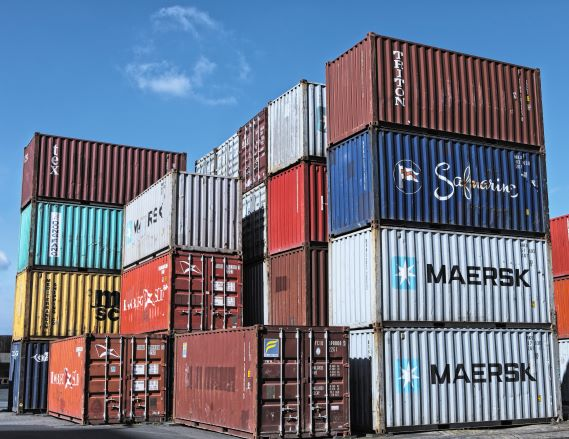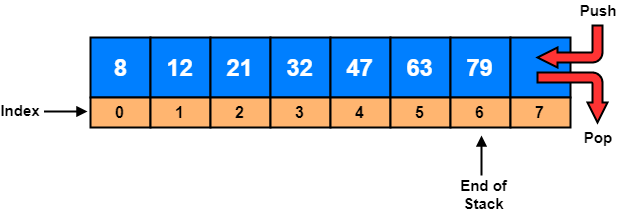# Stacks and Queues

Stacks and Queues are two fundamental data structures often used in Computer Science.

Stacks, like the name suggests, follow Last-In-First-Out (LIFO) principle while Queues work with first come first served approach, in other words with First-In-First-Out (FIFO) principle.

## StacksLet’s imagine a stack of cargo containers where new containers are added to the top of the stack. Since they are very heavy, only the topmost cargo container can be moved (LIFO). The topmost containers must be removed one by one, to be able to reach the other cargo containers down in the stack.

To implement a stack, we need two basic operations: `push()` and `pop()`

`push()` operation appends an element on top of the stack and `pop()` operation removes an element from the top of the stack.Unlike lists or arrays, stacks do not allow random access to the objects they contain but insert (`push`) and delete (`pop`) operations are very fast with O(1) time complexity.

There are two ways to implement stacks in Python; with using lists1 and with using collections.deque2 class.

### Implementation of Stacks with Using Lists

``````class Stack:
def __init__(self):
self.stack = []

def push(self, val):
return self.stack.append(val)

def pop(self):
if self.is_empty():
return None
else:
return self.stack.pop()

def size(self):
return len(self.stack)

def is_empty(self):
return self.size() == 0
``````

### Implementation of Stacks with Using collections.deque class

``````from collections import deque

class Stack:
def __init__(self):
self.stack = deque()

def push(self, val):
return self.stack.append(val)

def pop(self):
if self.is_empty():
return None
else:
return self.stack.pop()

def size(self):
return len(self.stack)

def is_empty(self):
return self.size() == 0
``````

## Queues

The simplest analogy of a queue data structure is, the line of people that forms in front of a supermarket checkout counter. The first person to arrive at that queue will be the first person to be done with it (FIFO). New additions to the line are made to the back of the queue while removal happens in the front of the queue when the first person pays for her groceries and take them to her home.To implement a queue, we again need two basic operations: `enqueue()` and `dequeue()`

`enqueue()` operation appends an element to the end of the queue and `dequeue()` operation removes an element from the beginning of the queue.

Unlike lists or arrays, queues do not allow random access to the objects they contain but insert (`enqueue`) and delete (`dequeue`) operations are very fast with O(1) time complexity.

### Implementation of Queues

``````from collections import deque

class Queue:
def __init__(self):
self.queue = deque()

def enqueue(self, val):
return self.queue.append(val)

def dequeue(self):
if self.is_empty():
return None
else:
return self.queue.popleft()

def size(self):
return len(self.queue)

def is_empty(self):
return self.size() == 0
``````
1. Python Official Documentation, Using Lists as Stacks
2. Python Official Documentation, collections.deque class